# Python TensorFlow，卷积神经网络(CNN)，手动实现卷积神经网络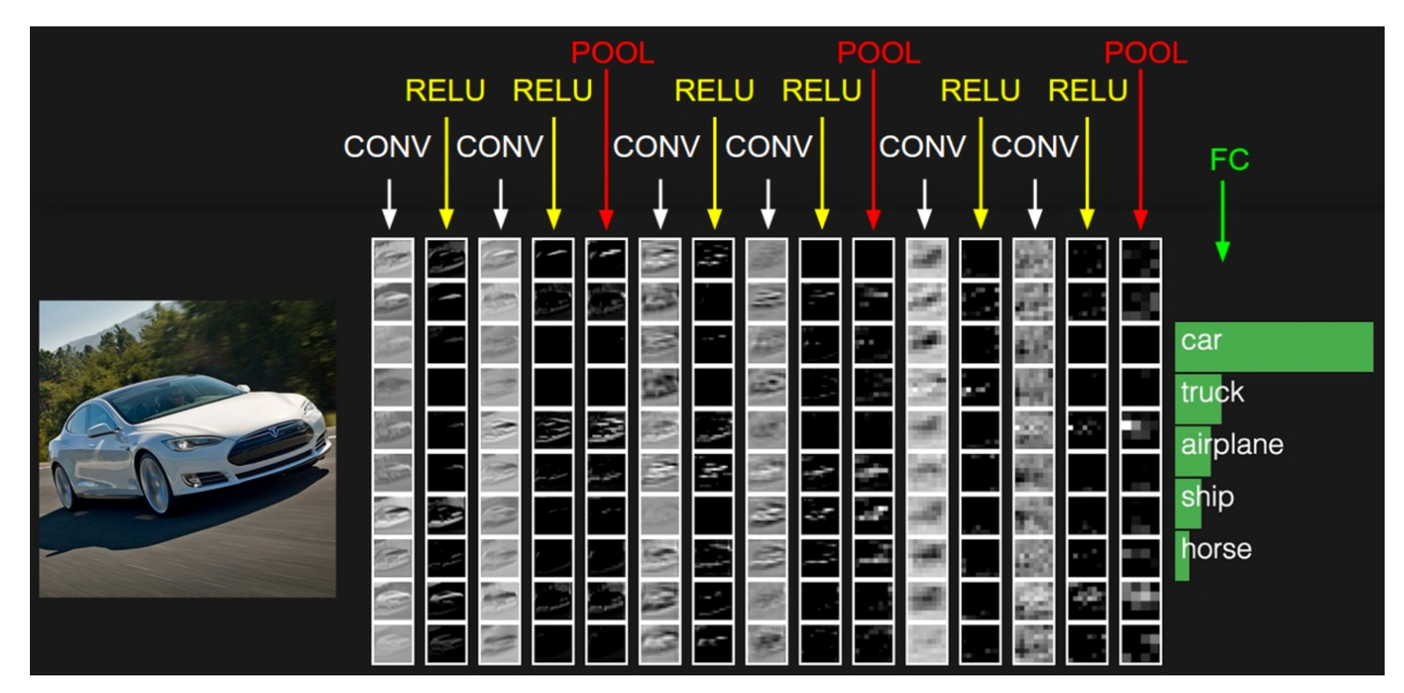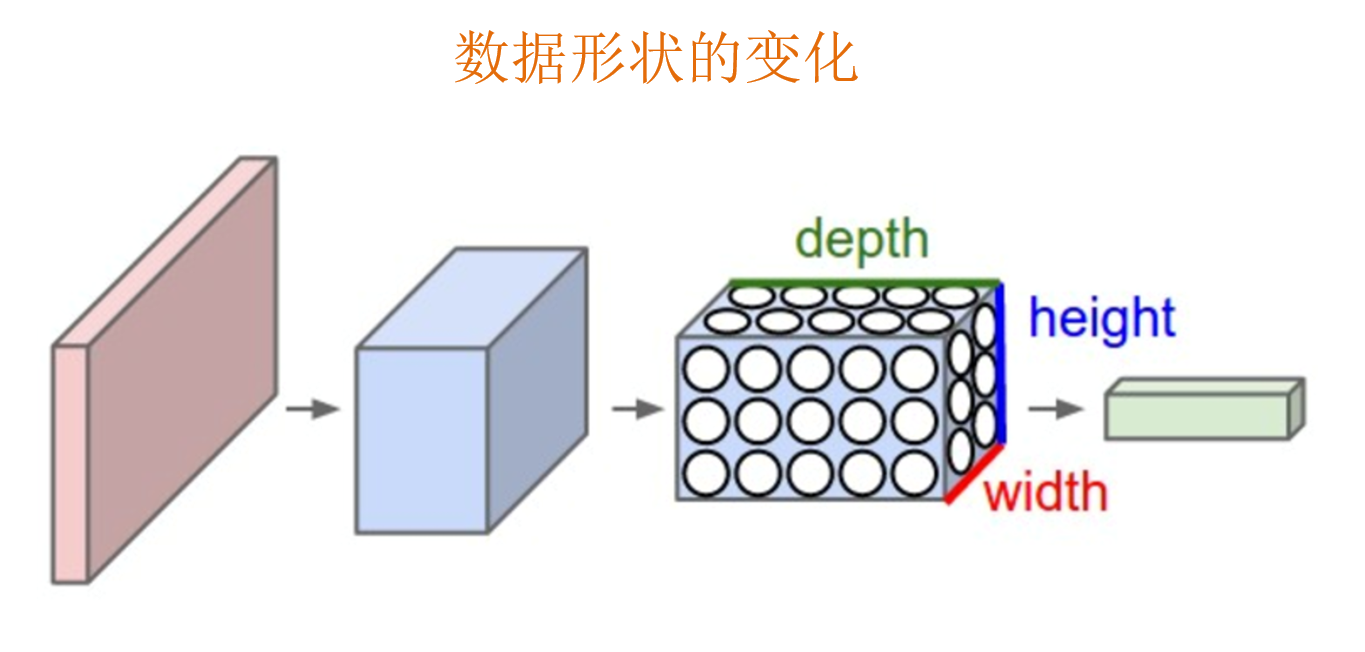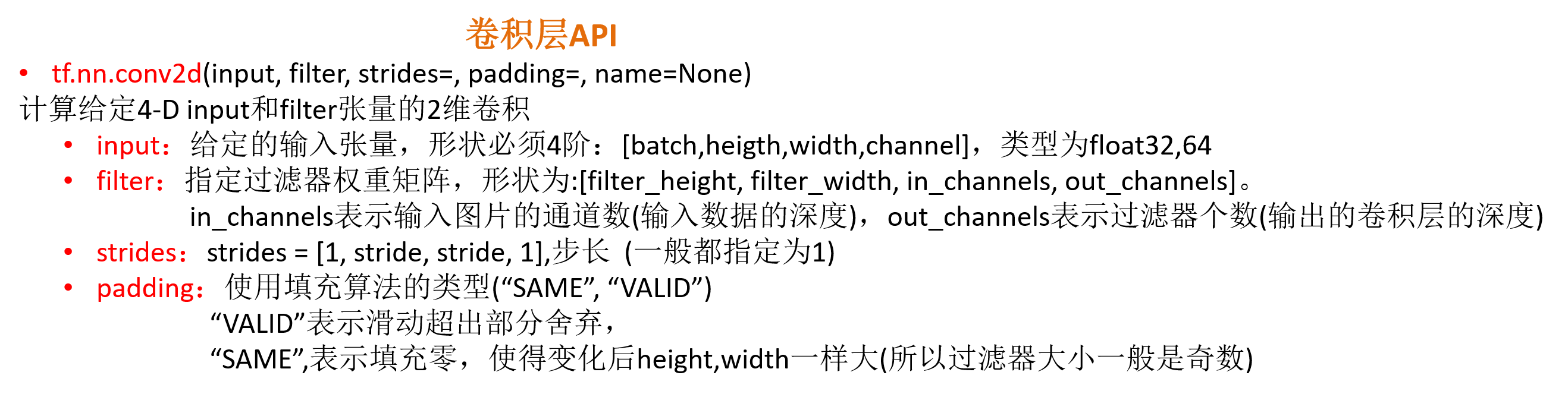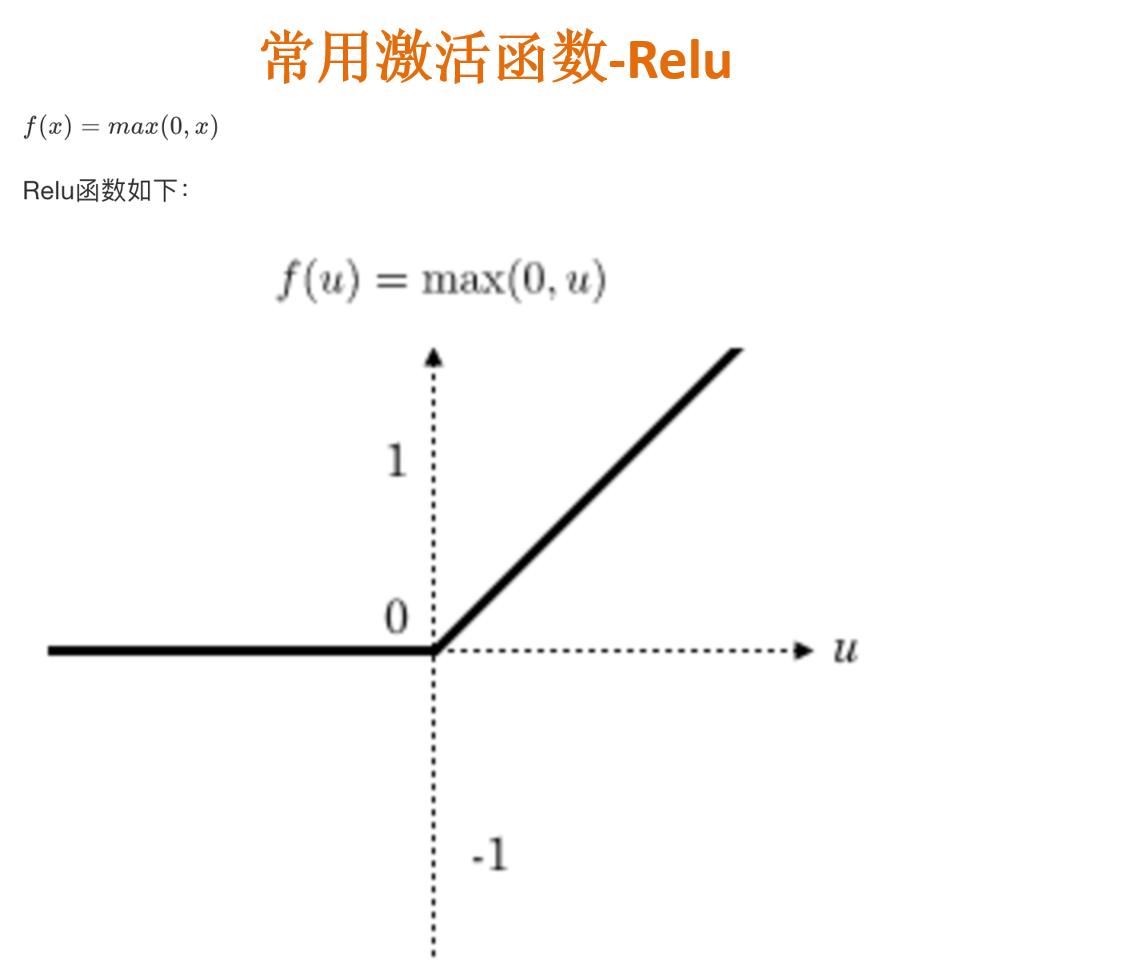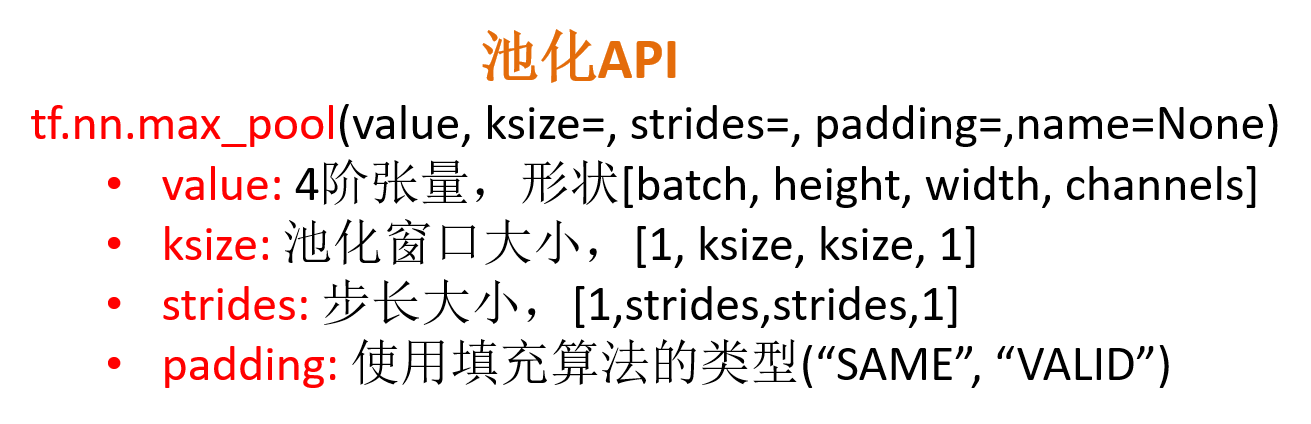### demo.py（手动实现一个卷积神经网络，预测手写数字图片）：

import tensorflow as tf
from tensorflow.examples.tutorials.mnist import input_data
# from tensorflow.contrib.slim.python.slim.nets.inception_v3 import inception_v3_base

# 读取数据
# TensorFlow自带的数据集(手写数字图片，55000个训练样本，10000个测试样本，图片尺寸28*28=784)
mnist = input_data.read_data_sets("./data/mnist/", one_hot=True)
# mnist.train.images  图片数据
# mnist.train.labels  目标标签(0-9,10个目标类别)
# mnist.train.next_batch(100)  可以批量获取数据

# 自定义的卷积神经网络模型

# 1、准备输入数据的占位符(与输入的真实数据的形状对应) x [None, 784]  y_true [None, 10](one-hot编码)  图片大小28*28=784
with tf.variable_scope("data"):
x = tf.placeholder(tf.float32, [None, 784])  # 图片大小28*28=784
y_true = tf.placeholder(tf.int32, [None, 10])  # 目标类别：10个类别。 one-hot编码形式表示
# 对x进行形状的改变(变成4阶张量) [None, 784]--->[None, 28, 28, 1]
x_reshape = tf.reshape(x, [-1, 28, 28, 1])  # 1个通道

# 2、图片数据-->卷积层-->激活函数-->池化层
with tf.variable_scope("conv_1"):
# 随机初始化卷积核的矩阵权重。  卷积核大小:5*5*1(1个通道,与输入图片通道数一致) 32个卷积核(决定卷积后形状的深度)
weight_conv_1 = tf.Variable(tf.random_normal(shape=[5, 5, 1, 32], mean=0.0, stddev=1.0))
# 初始化偏置。
bias_conv_1 = tf.Variable(tf.constant(0.0, shape=))  # 32个偏置

# 卷积、激活函数。 输入的张量x_reshape必须四阶   [None, 28, 28, 1]---> [None, 28, 28, 32]
x_relu_1 = tf.nn.relu(tf.nn.conv2d(x_reshape, weight_conv_1, strides=[1, 1, 1, 1], padding="SAME") + bias_conv_1)

# 池化。 窗口大小:2*2；步长:2    [None, 28, 28, 32]--->[None, 14, 14, 32]  池化只会改变形状的宽高,不会改变深度。
x_pool_1 = tf.nn.max_pool(x_relu_1, ksize=[1, 2, 2, 1], strides=[1, 2, 2, 1], padding="SAME")

# 3、再次卷积(深层神经网络)。 卷积层-->激活函数-->池化层
with tf.variable_scope("conv_2"):
# 随机初始化卷积核的矩阵权重。  卷积核大小:5*5*32  64个卷积核
weight_conv_2 = tf.Variable(tf.random_normal(shape=[5, 5, 32, 64], mean=0.0, stddev=1.0))
# 初始化偏置。
bias_conv_2 = tf.Variable(tf.constant(0.0, shape=))  # 64个偏置

# 卷积、激活函数。  [None, 14, 14, 32]---> [None, 14, 14, 64]  padding="SAME"：卷积只会改变形状的深度,不会改变宽高
x_relu_2 = tf.nn.relu(tf.nn.conv2d(x_pool_1, weight_conv_2, strides=[1, 1, 1, 1], padding="SAME") + bias_conv_2)

# 池化。 窗口大小:2*2；步长:2   [None, 14, 14, 64]--->[None, 7, 7, 64]  池化只会改变形状的宽高,不会改变深度。
x_pool_2 = tf.nn.max_pool(x_relu_2, ksize=[1, 2, 2, 1], strides=[1, 2, 2, 1], padding="SAME")

# 4、全连接层。  矩阵乘法：[None, 7, 7, 64]--->[None, 7*7*64]*[7*7*64, 10] +  = [None, 10] (目标类别数10,one-hot编码)
with tf.variable_scope("fc"):
# 随机初始化全连接的权重矩阵。  10表示目标类别数。
w_fc = tf.Variable(tf.random_normal(shape=[7*7*64, 10], mean=0.0, stddev=1.0))
# 初始化偏置。
b_fc = tf.Variable(tf.constant(0.0, shape=))

# 修改形状。  4阶--->2阶  [None, 7, 7, 64]--->[None, 7*7*64]
fc_reshape = tf.reshape(x_pool_2, [-1, 7*7*64])

# 进行矩阵运算得出每个样本的10个目标类别结果(可以通过softmax函数转换成10个目标类别的概率，最大的概率即为预测类别)
y_predict = tf.matmul(fc_reshape, w_fc) + b_fc

# 卷积神经网络模型定义结束

# 进行交叉熵损失计算
# 1、求出所有样本的交叉熵损失，然后求平均值
with tf.variable_scope("soft_cross"):
# 求平均交叉熵损失。  y_predict是没有经过softmax函数处理的预测结果。  y_true是真实的目标类别结果(one-hot编码)
loss = tf.reduce_mean(tf.nn.softmax_cross_entropy_with_logits(labels=y_true, logits=y_predict))

# 2、梯度下降优化损失
with tf.variable_scope("optimizer"):
train_op = tf.train.GradientDescentOptimizer(0.0001).minimize(loss)  # 0.0001表示学习率。(学习率并不是一个超参数)(深层神经网络的学习率一般设很小)

# 3、计算准确率
with tf.variable_scope("acc"):
equal_list = tf.equal(tf.argmax(y_true, 1), tf.argmax(y_predict, 1))  # y_true和y_predict形状：[None, 10] （one-hot编码）
# tf.argmax()返回最大值的下标,第二个参数1表示坐标轴(每一行的最大值)
# tf.equal()判断是否相等，返回0、1组成的张量(1表示相等,0表示不相等)

# tf.reduce_mean()计算平均值。 equal_list：None个样本[1, 0, 1, 0, 1, 1,..........]
accuracy = tf.reduce_mean(tf.cast(equal_list, tf.float32))

# 定义一个初始化变量的op
init_op = tf.global_variables_initializer()

# 开启会话运行
with tf.Session() as sess:
# 初始化变量
sess.run(init_op)

# 迭代训练，更新参数 (迭代1000次)
for i in range(1000):

# 取出训练集的特征值和目标值 (每次迭代取出50个样本)
mnist_x, mnist_y = mnist.train.next_batch(50)

# 运行train_op训练优化 (feed_dict：用实时的训练数据填充占位符)
sess.run(train_op, feed_dict={x: mnist_x, y_true: mnist_y})

print("训练第%d步,准确率为:%f" % (i, sess.run(accuracy, feed_dict={x: mnist_x, y_true: mnist_y})))